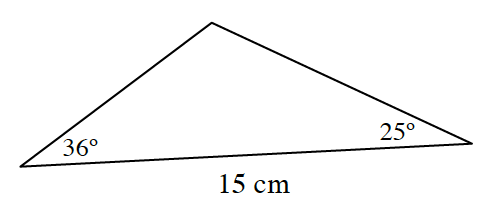### Home > PC3 > Chapter 9 > Lesson 9.1.4 > Problem9-84

9-84.

Calculate the area of the triangle shown at right. Homework Help ✎

Use the Law of Cosines to determine the length of one of the missing sides.

area $=\frac{1}{2}ab\sin(θ)$
where $θ$ is the angle between sides $a$ and $b$